# Explain the transformation in general

Publish On: 2019-03-24

Total Post: 559

# Mjay Jollay

Total Post: 0

## ANS: Explain the transformation in general

Let ƒ(x) = 0 be a given equation whose roots are connected by the given relation F(x, y) = 0, known as the equation of transformation. Then the transformed equation Ø(y) = 0 is obtained by eliminating x from ƒ(x) = 0 and F(x, y) = 0.

Example: if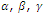are the roots of the equation

x3 – px2 + qx = 0,

form an equation whose roots are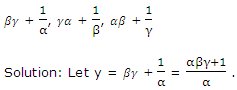Now,are the roots of the equation

x3 – px2 + qx – r = 0                       (1)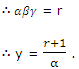Since x =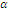is the root of the equation (1), hence the relation of transformation is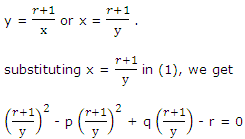Or, ry3 – q(1 + r)y2 + p(1 + r)2y – (1 + r)3 = 0

Or, rx3 – q(1 + r)x2 + p(1 + r)x = (1 + r)3 = 0

is the required equation.